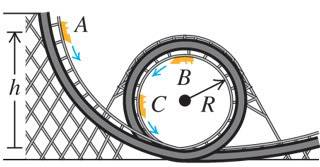# Compute the tangential acceleration

• cycam

## Homework Statement

If the car starts at height h = 60.0 m and the radius is R = 15.0 m, compute the tangential acceleration of the passengers when the car is at point C, which is at the end of a horizontal diameter.
Take the free fall acceleration to be g = 9.80 m/s^2.## The Attempt at a Solution

Have tried a couple of solutions but have limited chances left. Don't want to get it wrong, any ideas?

Got it, nevermind!

BUMP

How did you figure it out? I'm completely lost.